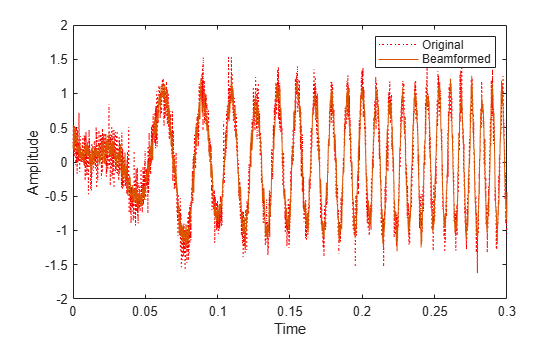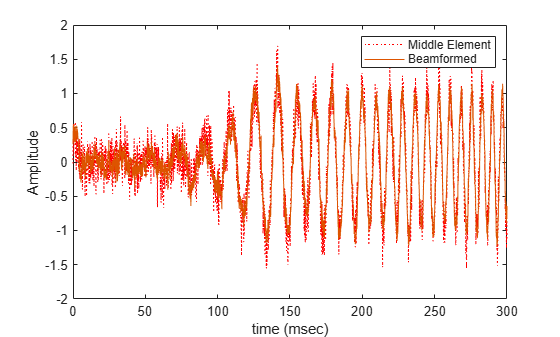# phased.FrostBeamformer

Frost beamformer

## Description

The `phased.FrostBeamformer` object implements a Frost beamformer. A Frost beamformer consists of a time-domain MVDR beamformer combined with a bank of FIR filters. The beamformer steers the beam towards a given direction while the FIR filters preserve the input signal power.

To compute the beamformed signal:

1. Create the `phased.FrostBeamformer` object and set its properties.

2. Call the object with arguments, as if it were a function.

## Creation

### Syntax

``beamformer = phased.FrostBeamformer``
``beamformer = phased.FrostBeamformer(Name,Value)``

### Description

````beamformer = phased.FrostBeamformer` creates a Frost beamformer System object™, `beamformer`, with default property values.```
````beamformer = phased.FrostBeamformer(Name,Value)` creates a Frost beamformer object, `beamformer`, with each specified property Name set to the specified Value. You can specify additional name-value pair arguments in any order as (`Name1`,`Value1`,...,`NameN`,`ValueN`). Enclose each property name in single quotes.Example: ```beamformer = phased.FrostBeamformer('SensorArray',phased.ULA('NumElements',20),'SampleRate',300e3)``` sets the sensor array to a uniform linear array (ULA) with default ULA property values except for the number of elements. The beamformer has a sample rate of 300 kHz.```

## Properties

expand all

Unless otherwise indicated, properties are nontunable, which means you cannot change their values after calling the object. Objects lock when you call them, and the `release` function unlocks them.

If a property is tunable, you can change its value at any time.

Sensor array, specified as a Phased Array System Toolbox array System object. The array cannot contain subarrays.

Example: `phased.URA`

Signal propagation speed, specified as a real-valued positive scalar. Units are in meters per second. The default propagation speed is the value returned by `physconst('LightSpeed')`.

Example: `3e8`

Data Types: `single` | `double`

Sample rate of signal, specified as a positive scalar. Units are in Hz. The System object uses this quantity to calculate the propagation delay in units of samples.

Example: `1e6`

Data Types: `single` | `double`

Length of FIR filter for each sensor element, specified as a positive integer.

Example: `7`

Data Types: `single` | `double`

Diagonal loading factor, specified as a nonnegative scalar. Diagonal loading is a technique used to achieve robust beamforming performance, especially when the sample size is small. A small sample size can lead to an inaccurate estimate of the covariance matrix. Diagonal loading also provides robustness due to steering vector errors. The diagonal loading technique adds a positive scalar multiple of the identity matrix to the sample covariance matrix.

Tunable: Yes

Data Types: `single` | `double`

Enable training data input, specified as `false` or `true`. When you set this property to `true`, use the training data input argument, `XT`, when running the object. Set this property to `false` to use the input data, `X`, as the training data.

Data Types: `logical`

Source of beamforming direction, specified as `'Property'` or ```'Input port'```. Specify whether the beamforming direction comes from the `Direction` property of this object or from the input argument, `ANG`. Values of this property are:

 `'Property'` Specify the beamforming direction using the `Direction` property. `'Input port'` Specify the beamforming direction using the input argument, `ANG`.

Data Types: `char`

Beamforming directions, specified as a real-valued 2-by-1 vector or a real-valued 2-by-L matrix. For a matrix, each column specifies a different beamforming direction. Each column has the form `[AzimuthAngle;ElevationAngle]`. Azimuth angles must lie between –180° and 180° and elevation angles must lie between –90° and 90°. All angles are defined with respect to the local coordinate system of the array. Units are in degrees.

Example: `[40;30]`

#### Dependencies

To enable this property, set the `DirectionSource` property to `'Property'`.

Data Types: `single` | `double`

Enable the output of beamforming weights, specified as `false` or `true`. To obtain the beamforming weights, set this property to `true` and use the corresponding output argument, `W`. If you do not want to obtain the weights, set this property to `false`.

Data Types: `logical`

## Usage

### Syntax

``Y = beamformer(X)``
``Y = beamformer(X,XT)``
``Y = beamformer(X,ANG)``
``Y = beamformer(X,XT,ANG)``
``[Y,W] = beamformer(___)``

### Description

example

````Y = beamformer(X)` performs Frost beamforming on the input, `X`, and returns the beamformed output, `Y`. This syntax uses the input data, `X`, as training samples to calculate the beamforming weights.```
````Y = beamformer(X,XT)` uses `XT` as training data to calculate the beamforming weights. To use this syntax, set the TrainingInputPort property to `true`.```
````Y = beamformer(X,ANG)` uses `ANG` as the beamforming direction. To use this syntax, set the DirectionSource property to `'Input port'`.```
````Y = beamformer(X,XT,ANG)` combines all input arguments. To use this syntax, set the TrainingInputPort property to `true` and set the DirectionSource property to `'Input port'`.```

example

````[Y,W] = beamformer(___)` returns the beamforming weights, `W`. To use this syntax, set the WeightsOutputPort property to `true`.```

### Input Arguments

expand all

Input signal, specified as a complex-valued M-by-N matrix. M is the signal length and N is the number of array elements specified in the SensorArray property. M must be larger than the length of the filter specified by the FilterLength property.

The size of the first dimension of the input matrix can vary to simulate a changing signal length. A size change can occur, for example, in the case of a pulse waveform with variable pulse repetition frequency.

Data Types: `single` | `double`
Complex Number Support: Yes

Training data, specified as a complex-valued M-by-N matrix. M and N are equal to the values for `X`.

The size of the first dimension of the input matrix can vary to simulate a changing signal length. A size change can occur, for example, in the case of a pulse waveform with variable pulse repetition frequency.

Example: `[1 0.5 2.6; 2 -0.2 0; 3 -2 -1]`

#### Dependencies

To enable this argument, set the TrainingInputPort property to `true`.

Data Types: `single` | `double`
Complex Number Support: Yes

Beamforming directions, specified as a real-valued 2-by-1 column vector The vector has the form `[AzimuthAngle;ElevationAngle]`. Units are in degrees. The azimuth angle must lie between –180° and 180°, and the elevation angle must lie between –90° and 90°.

Example: `[40;10]`

#### Dependencies

To enable this argument, set the DirectionSource property to `'Input port'`.

Data Types: `double`

### Output Arguments

expand all

Beamformed output, returned as a complex-valued 1-by-Mvector, where M is the number of rows of the input `X`.

Data Types: `single` | `double`
Complex Number Support: Yes

Beamforming weights, returned as a complex-valued L-by-1 vector where L is the number of degrees of freedom of the beamformer. The number of degrees of freedom is given by the product of the number of elements specified by the SensorArray property and the FIR filter length specified by FilterLength property.

#### Dependencies

To enable this output, set the WeightsOutputPort property to `true`.

Data Types: `single` | `double`
Complex Number Support: Yes

## Object Functions

To use an object function, specify the System object as the first input argument. For example, to release system resources of a System object named `obj`, use this syntax:

`release(obj)`

expand all

 `step` Run System object algorithm `release` Release resources and allow changes to System object property values and input characteristics `reset` Reset internal states of System object

## Examples

collapse all

Apply Frost beamforming to an 11-element acoustic ULA array. The incident angle of the incoming signal is -50 degrees in azimuth and 30 degrees in elevation. The speed of sound in air is assumed to be 340 m/sec. The signal has added Gaussian white noise.

Simulate the signal.

```array = phased.ULA('NumElements',11,'ElementSpacing',0.04); array.Element.FrequencyRange = [20 20000]; fs = 8e3; t = 0:1/fs:0.3; x = chirp(t,0,1,500); c = 340; collector = phased.WidebandCollector('Sensor',array,... 'PropagationSpeed',c,'SampleRate',fs,... 'ModulatedInput',false,'NumSubbands',8192); incidentAngle = [-50;30]; x = collector(x.',incidentAngle); noise = 0.2*randn(size(x)); rx = x + noise;```

Beamform the signal.

```beamformer = phased.FrostBeamformer('SensorArray',array,... 'PropagationSpeed',c,'SampleRate',fs,... 'Direction',incidentAngle,'FilterLength',5); y = beamformer(rx);```

Plot the beamformed output.

```plot(t,rx(:,6),'r:',t,y) xlabel('Time') ylabel('Amplitude') legend('Original','Beamformed')```Find the beamformer weights of a Frost beamforming applied to signals received at a 7-element acoustic ULA array. The incident angle of the incoming signal is $-2{0}^{\circ }$ in azimuth and $3{0}^{\circ }$ in elevation. The signal has added Gaussian white noise. The speed of sound in air is assumed to be 340 m/s. Use a filter length of 15.

First, create the signal.

```numelements = 7; element = phased.OmnidirectionalMicrophoneElement('FrequencyRange',[50,10000]); array = phased.ULA('Element',element,'NumElements',numelements,'ElementSpacing',0.04); fs = 8e3; t = 0:1/fs:0.3; x = chirp(t,0,1,500); c = 340.0; collector = phased.WidebandCollector('Sensor',array,... 'PropagationSpeed',c,'SampleRate',fs,... 'ModulatedInput',false,'NumSubbands',8192); incidentAngle = [-20;30]; x = collector(x.',incidentAngle); noise = 0.2*randn(size(x)); rx = x + noise;```

Create a beamformer with a filter length of 15. Then, beamform the arriving signal and obtain the beamformer weights.

```filterlength = 15; beamformer = phased.FrostBeamformer('SensorArray',array, ... 'PropagationSpeed',c,'SampleRate',fs,'WeightsOutputPort',true, ... 'Direction',incidentAngle,'FilterLength',filterlength); [y,wt] = beamformer(rx); size(wt)```
```ans = 1×2 105 1 ```

There are 7*15 = 105 weights computed as expected.

Compare the beamformed output with the signal arriving at the middle element of the array.

```plot(1000*t,rx(:,4),'r:',1000*t,y) xlabel('time (msec)') ylabel('Amplitude') legend('Middle Element','Beamformed')```## Algorithms

`phased.FrostBeamformer` uses a beamforming algorithm proposed by Frost. It can be considered the time-domain counterpart of the minimum variance distortionless response (MVDR) beamformer. The algorithm does the following:

1. Steers the array to the beamforming direction.

2. Applies an FIR filter to the output of each sensor to achieve the distortionless response constraint. The filter is specific to each sensor.

3. This System object supports single and double precision for input data, properties, and arguments. If the input data `X` is single precision, the output data is single precision. If the input data `X` is double precision, the output data is double precision. The precision of the output is independent of the precision of the properties and other arguments.